Courses

# RD Sharma Solutions Ex-13.4, Linear Equation In Two Variables, Class 9, Maths Class 9 Notes | EduRev

## Class 9 : RD Sharma Solutions Ex-13.4, Linear Equation In Two Variables, Class 9, Maths Class 9 Notes | EduRev

The document RD Sharma Solutions Ex-13.4, Linear Equation In Two Variables, Class 9, Maths Class 9 Notes | EduRev is a part of the Class 9 Course RD Sharma Solutions for Class 9 Mathematics.
All you need of Class 9 at this link: Class 9

Q1: Give the geometric representations of the following equations

(a) on the number line

(b) on the Cartesian plane:

(i) x = 2
(ii) y + 3 = 0
(iii) y = 3
(iv) 2x + 9 = 0
(v) 3x – 5 = 0

Ans :

(i) We are given, x = 2

The representation of the solution on the number line, when given equation is treated as an equation in one variable.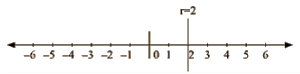The representation of the solution on the Cartesian plane, it is a line parallel to y axis passing througl-the point (2, 0) is shown below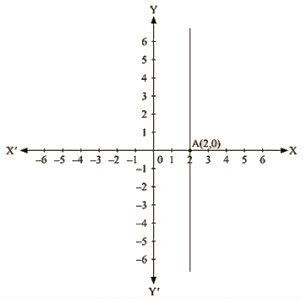(ii)  We are given, y + 3 = 0

We get, Y = -3

The representation of the solution on the number line, when given equation is treated as an equation in one variable.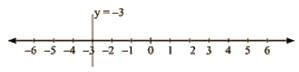The representation of the solution on the Cartesian plane, it is a line parallel to x axis passing through the point A(0, —3) is shown below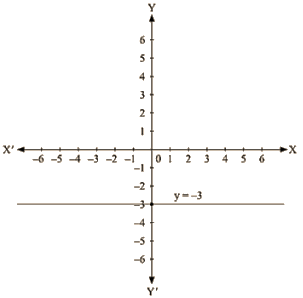(iii) we are given. y = 3

The representation of the solution on the number line. when given equation is treated as an equation in one variable.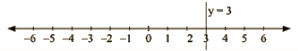The representation of the solution on the Cartesian plane, it is a line parallel to x axis passing through the point (0, 3) is shown below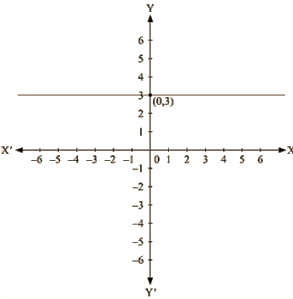(iv) We are given, 2x +9 = 0

We get,  2x = -9 The representation of the solution on the number line, when given equation is treated as an equation in one variable.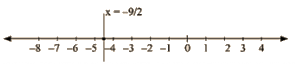The representation of the solution on the Cartesian plane, it is a line parallel to y axis passing through the point ( -9/2,0) is shown below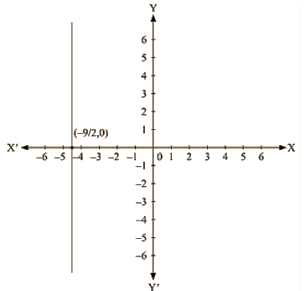(v) We are given, 3x —5 = 0

We get, 5 x = 3 The representation of the solution on the number line, when given equation is treated as an equation in one variable.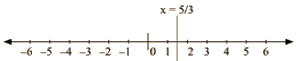The representation of the solution on the Cartesian plane, it is a line parallel to y axis passing througl-the point (5,0) is shown below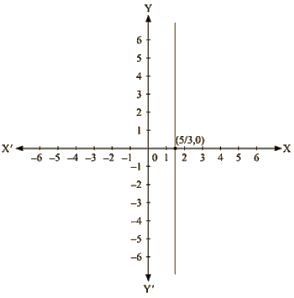Q 2 : Give the geometrical representation of 2x + 13 = 0 as an equation in

(i) one variable
(ii) two variables

Ans:

We are given,

2x +13 = 0

We get,

2x = -13

x = -13/2

The representation of the solution on the number line, when given equation is treated as an equation in one variable.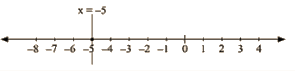The representation of the solution on the Cartesian plane, it is a line parallel to y axis passing through the point (-13/2 , 0) is shown below.

Q3:. Solve the equation 3x + 2 = x – 8, and represent the solution on

(i) the number line
(ii) the Cartesian plane.

Ans : We are given,

3x + 2 = x – 8

we get,

3x – x =  -8 – 2

2x = -10

x = -5

The representation of the solution on the number line, when given equation is treated as an equation in one variable.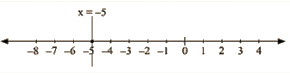The representation Of the solution on the Cartesian plane, it is a line parallel to y axis passing through the point (-5, 0) is shown below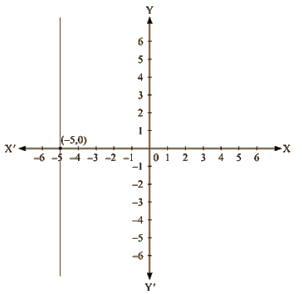Q 4:  Write the equation of the line that is parallel to x-axis and passing through the points

(i) (0,3)
(ii) (0, – 4)
(iii) (2,-5)
(iv) (3,4)

Ans:

(i) We are given the co-ordinates of the Cartesian plane at (0,3).

For the equation of the line parallel to x axis, we assume the equation as a one variable equation independent of x containing y equal to 3.

We get the equation as y = 3

(ii) We are given the co-ordinates of the Cartesian plane at (0,-4).

For the equation of the line parallel to x axis, we assume the equation as a one variable equation Independent of x containing y equal to -4.

We get the equation as y = -4

(iii) We are given the co-ordinates of the Cartesian plane at (2,-5).

For the equation of the line parallel to x axis, we assume the equation as a one variable equation independent of x containing y equal to -5.

We get the equation as y = -5

(iv) We are given the co-ordinates of the Cartesian plane at (3,4).

For the equation of the line parallel to x axis, we assume the equation as a one variable equation independent of x containing y equal to 4.

We get the equation as

y = 4

Q 5 : Write the equation of the line that is parallel to y-axis and passing through the Points

(i) (4,0)
(ii) (-2,0)
(iii) (3,5)
(iv) (- 4, – 3)

Ans:

(i) We are given the coordinates of the Cartesian plane at (4,0)-

For the equation of the line parallel to y axis ,we assume the equation as a one variable equation independent of y containing x equal to 4

We get the equation as y = 3

(ii) We are given the coordinates of the Cartesian plane at (-2,0) –

For the equation of the line parallel to y axis, we assume the equation as a one variable equation independent of y containing x equal to -2

We get the equation as y = -4

(iii) We are given the coordinates of the Cartesian plane at (3,5)-

For the equation of the line parallel to y axis, we assume the equation as a one variable equation independent of y containing x equal to 3

We get the equation as y = -5

(iv) We are given the coordinates of the Cartesian plane at (-4,-3)-

For the equation of the line parallel to y axis, we assume the equation as a one variable equation independent of y containing x equal to -4

We get the equation as y = 4

Offer running on EduRev: Apply code STAYHOME200 to get INR 200 off on our premium plan EduRev Infinity!

91 docs

,

,

,

,

,

,

,

,

,

,

,

,

,

,

,

,

,

,

,

,

,

,

,

,

,

,

,

,

,

,

;